Next: The Role of the Positive Muon Up: Measuring the penetration depth with TF-SR Previous: Measuring the penetration depth with TF-SR

# The Field Distribution

The vortex lattice in a type-II superconductor results in a spatially varying magnetic field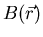inside the superconductor. Transverse -field muon spin rotation (TF-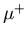SR) can be utilized to investigate the local magnetic field distribution function [72,77]: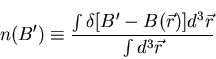(1)

The function n(B) denotes the field density. Specifically it is the probability that the magnitude of the magnetic field at a point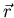inside the superconductor is B. Eq. (3.1) defines n(B) as the spatial average of a one-dimensional Dirac delta function. By definition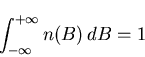(2)

The field distribution pertaining to a perfect vortex lattice free of distortions resembles that of Fig. 3.1. The magnetic lineshape exhibits van Hove singularities corresponding to:

A: the minimum value ofwhich occurs at the center of the vortex lattice.
B: the saddle point value ofwhich occurs midway between two vortices.
C: the maximum value ofwhich occurs at the vortex cores.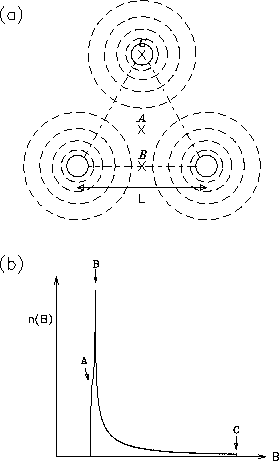The average local magnetic field strength inside the superconductor is denoted. The spatial average of B is just the first moment of n(B) :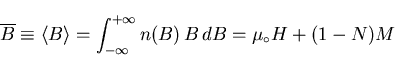(3)

where H is the magnitude of the externally applied field, N is the demagnetization factor (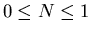) and M is the magnetization of the sample. The demagnetization factor N depends on the shape of the sample. The second moment of n(B) determines the width of the field distribution and is given by: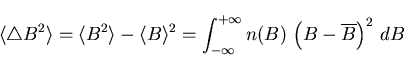(4)

If the applied field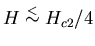then the London model is valid. At such fields the spacing L between adjacent vortices is large compared to the coherence length. In the London theory, the local magnetic field in an isotropic superconductor is given as :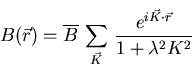(5)

where the sum extends over the reciprocal lattice vectors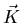of the vortex lattice. Combining (3.4) and (3.5), the second moment of n(B) for a triangular flux lattice as determined in the London picture is [32,68]: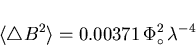(6)

It is clear from Eq. (3.6) that one can estimate the penetration depth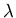from the second moment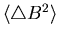, so thatis inherently related to the line width of the measured field distribution. Eq. (3.6) is valid at intermediate fields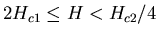where the second momentand henceis independent of the applied field [34,74]. At such fields the inter-vortex spacing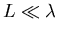. Rammer argues that in the case of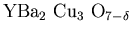, Eq. (3.6) is useful only for fields significantly smaller than 50kG (i.e. 5.0T), and above this significant deviations from the London model result rammer. For low fields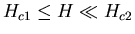, where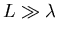and the vortices are well separated, the second moment as determined in the London limit is related to the average local fieldapproximately by :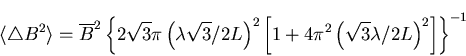(7)

This result was derived for a triangular flux-line lattice. According to Eq. (3.7), increasing the applied field in this region leads to a corresponding increase in the second moment. This statement of course assumes that the penetration depthis a field independent quantity.

At high fields the second moment decreases with increasing applied field Has the vortex cores start to overlap. The second moment at such fields can be estimated by using Abrikosov's numerical solution of the GL-equations for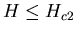: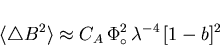(8)

where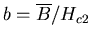and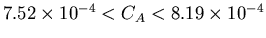, with the lower limit given by  and the upper limit given by . London theory is not valid in this high-field regime, where the inter-vortex spacing of the lattice is comparable to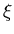[i.e.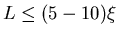] and the vortex cores start to overlap .

In London theory, whereis assumed to be zero, the local magnetic field in the center of a vortex is not finite. To get around this divergence, Brandt [34,72] approximates the vortex core by a gaussian function of width, which provides a dome-shaped peak for the field. An additional term (1-b) can be incorporated to roughly account for the field dependence of the superconducting order parameter . As in Eq. (3.8) this term is significant for applied fields close to the upper critical field Hc2, leading to a noticeable reduction in the width of the magnetic lineshape. Including this effect, the vortex core can be approximated by a gaussian function of width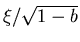. Thus, according to Brandt [34,72,76], one can modify Eq. (3.5) so that the local magnetic field of an isotropic superconductor for applied fields H < Hc2/4 is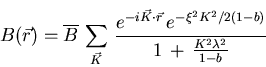(9)

The second exponential in Eq. (3.9) introduces an upper cutoff for the reciprocal lattice vectors at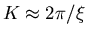. This value emerged from Brandt's numerical solution of the GL-equations [34,72]. The upper cutoff forleads to a finite value of the local field B at the vortex core. Fig. 3.2 illustrates the field distribution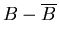as determined by Eq. (3.9) for the average internal magnetic fields=5kG and 15kG. As the field is increased, the distance between vortex cores decreases. Also the difference between the maximum and minimum field in the distribution decreases with increasing applied field. Fig. 3.3 shows the effects on the field distribution of increasing the magnetic penetration depth, while Fig. 3.4 illustrates the dependence on the GL-parameter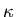.

The variation of the supercurrent density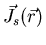with positionfrom the vortex centre can be determined by substitution of Eq. (3.9) into Eq. (2.3). Fig. 3.5 illustrates the dependence of the absolute value of the supercurrent density distribution on magnetic field. The supercurrent density is zero at the centre of a vortex core, corresponding to the maximum in the field distribution; Js rises steeply to its maximum value at the edge of the vortex core and then falls off exponentially. The singularity observed halfway between two vortices denotes a change in the flow direction of the supercurrents.

Fig. 3.8 shows the dependence of the average value of the supercurrent density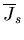on the average fieldfor. As the applied field (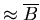) is increased above the lower critical field, the average supercurrent density rises steeply. This is because the supercurrents which screen the vortex cores have less room to spread out at higher fields when the cores are closer together. However, as shown in Fig. 3.8, the relationship is not linear. This is due to the overlapping of vortices, which tends to reduce. As the field is increased, the inter-vortex separation decreases. Eventually the overlapping of vortices dominates the contribution to the average supercurrent density and the curve turns over, so thatdecreases with further increase in the applied magnetic field. Interestingly, the turnover in Fig. 3.8 occurs around 50kG; the maximum field at which Rammer claims Eq. (3.6) is valid . The dependence of the supercurrent distribution onandcan be seen in Fig. 3.6 and Fig. 3.7, respectively.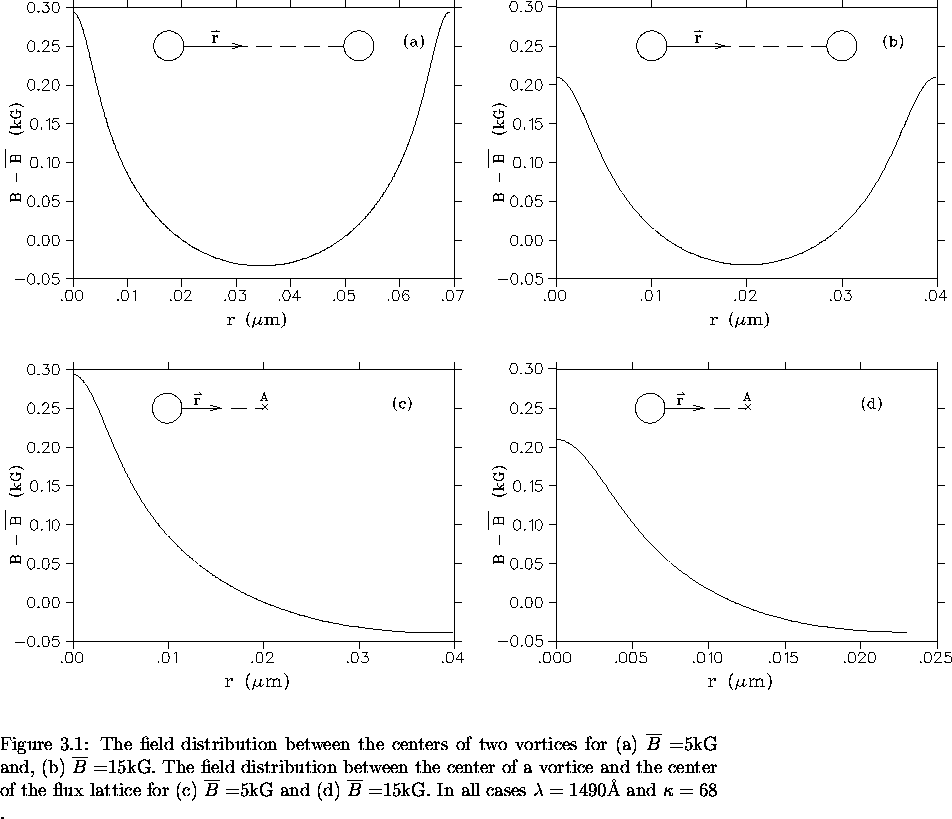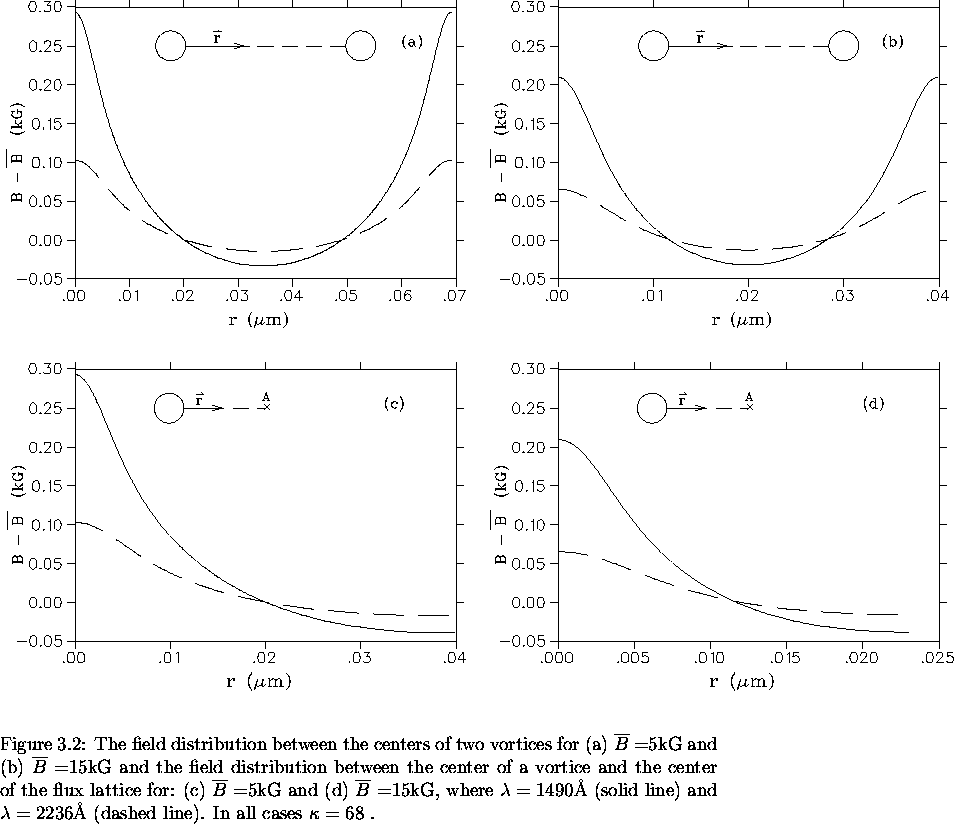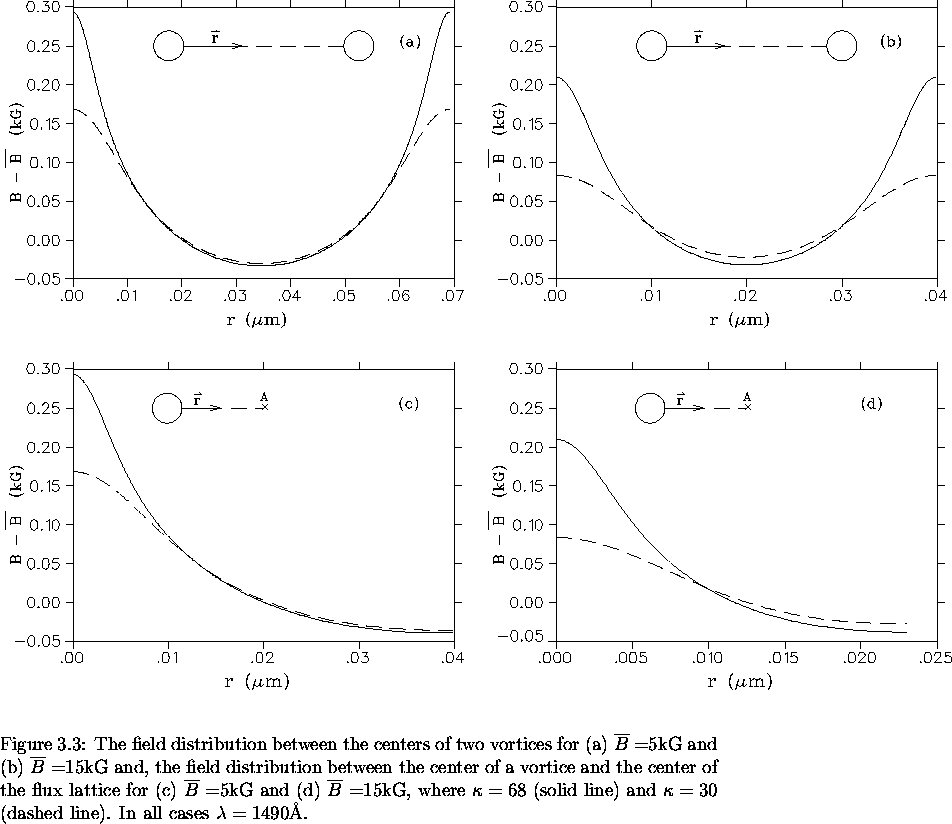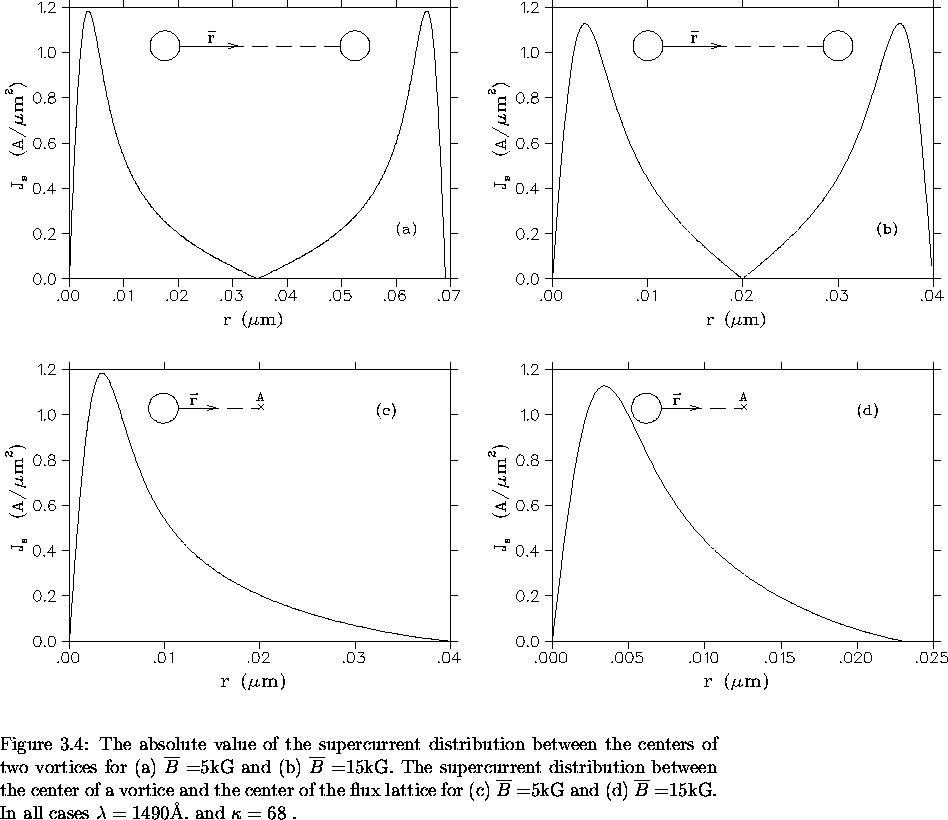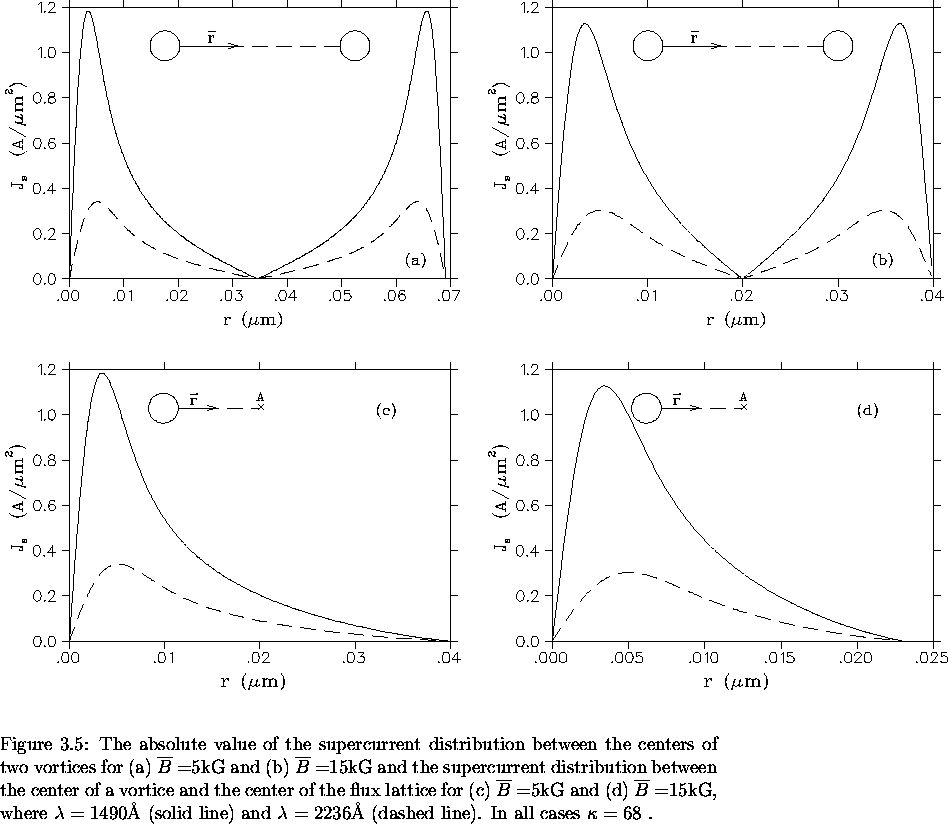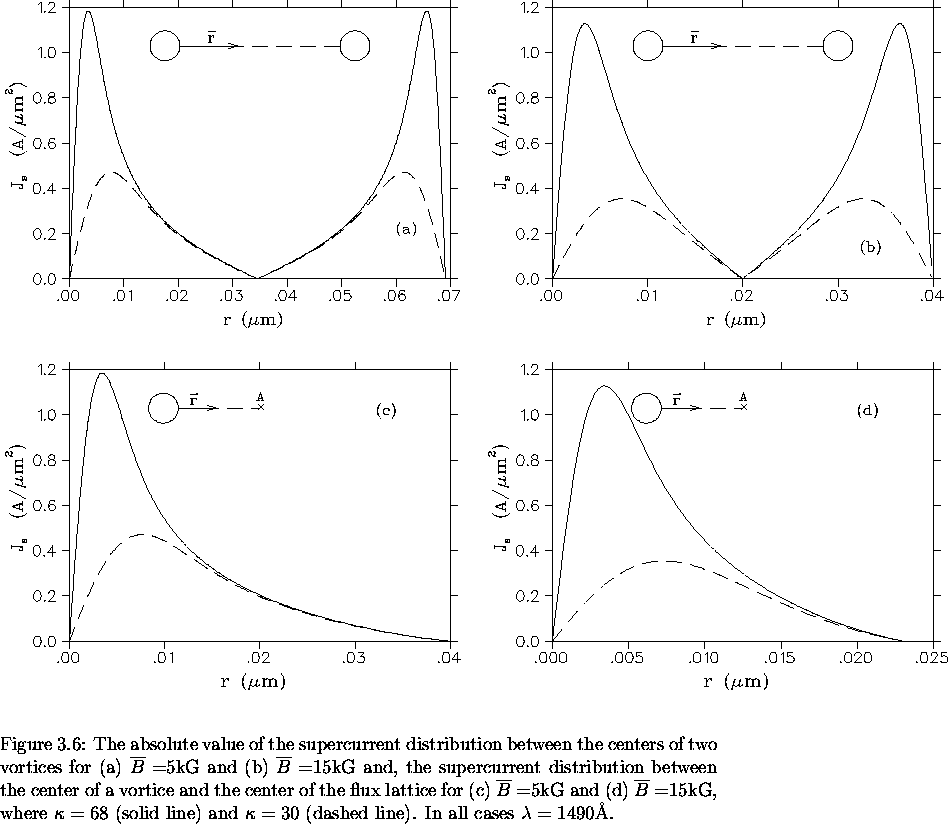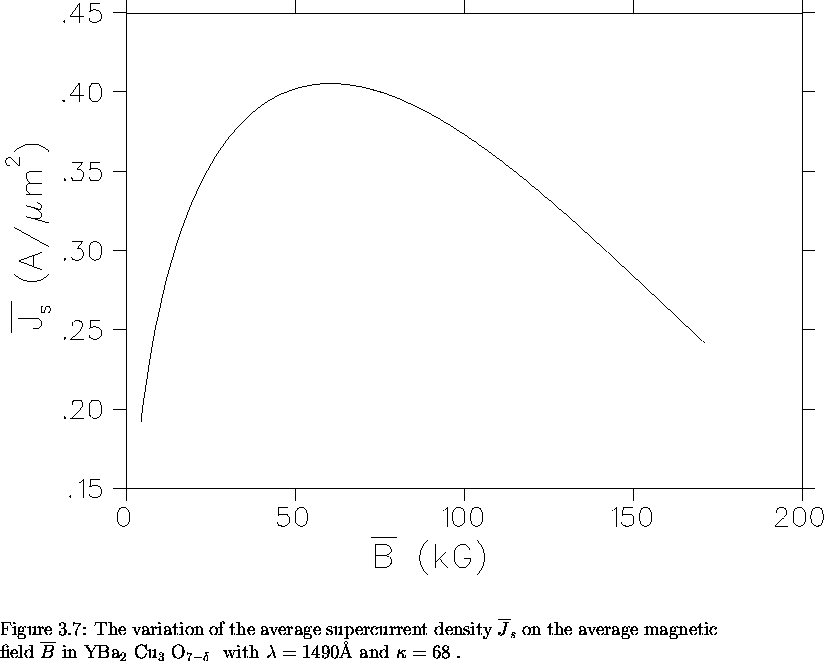As mentioned in the previous chapter, the orientation of the applied field with respect to the crystallographic axes of the superconductor is important. For an applied field parallel to the c-axis, the second moment of n(B) valid at intermediate fields can be written as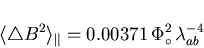(10)

and for an applied field parallel to the ab-plane,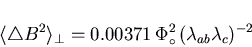(11)

In the latter case, the supercurrents flow across the ab-plane and along the c-axis. In fact, for the uniaxial anisotropic high-Tc superconductors, there exists a nonzero component of magnetic field transverse to the vortex axes, when the applied field is not directed along one of the principal axes in the ab-plane or along the c-axis . As the angle between the applied field and the crystallographic c-axis is increased, the vortex lattice distorts. The circular cross-section of an isolated vortex line associated with an applied field directed parallel to the c-axis (see Fig. 3.1) is replaced by an elliptical cross-section as shown in Fig. 3.9. This is a result of the anisotropy of the supercurrents. Correspondingly, the equilateral-triangular vortex lattice is stretched into an isosceles one.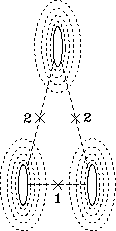The peak in the measuredSR field distribution n(B) will be split because now there are two types of saddle points. However, because the vortices themselves are stretched, the saddle points are comparable; so that experimentally, a broadening of the peak in n(B) may be observed, rather than a splitting. The width of the field distribution n(B), as evidenced by Eqs. (3.10) and (3.11), is of course influenced by the anisotropic distortions of the vortex lattice. However it is clear from Eq. (3.10), that by orientating the sample with its crystallographic c-axis parallel to the applied field, the problem becomes isotropic in nature. Of course this is only true if one is ignoring the smaller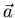-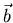anisotropy.Next: The Role of the Positive Muon Up: Measuring the penetration depth with TF-SR Previous: Measuring the penetration depth with TF-SR
Jess H. Brewer
2001-09-28